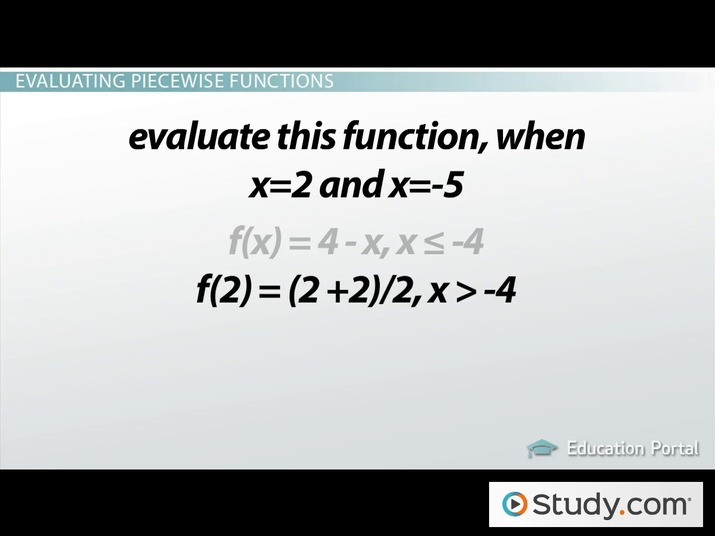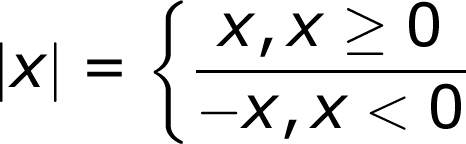# What are Piecewise Functions?

Lesson Transcript
Instructor: Jennifer Beddoe

Jennifer has an MS in Chemistry and a BS in Biological Sciences.

Piecewise functions have several parts, or pieces and are defined by a domain. Discover more about piecewise functions, some examples, how to evaluate them, and their application in the real world. Updated: 10/13/2021

When we talk about a person's function, we are talking about their job or role, the thing that they do. In math, a function is defined as a set of outputs related to specific inputs. This really just means that a function is an equation with a specific job to do. When you input a certain number into the equation, you will get a specific and unique number from the equation. Just like, for example, the function of the letter carrier. They get the mail (input) and deliver it to the proper address (output).An error occurred trying to load this video.

Try refreshing the page, or contact customer support.

Coming up next: How to Find the Domain of Piecewise Functions

### You're on a roll. Keep up the good work!

Replay
Your next lesson will play in 10 seconds
• 0:38 Specific Types of…
• 1:21 Examples of Piecewise…
• 2:30 Evaluating Piecewise Functions
• 4:10 Real World Piecewise…
• 6:28 Lesson Summary
Save Save

Want to watch this again later?

Timeline
Autoplay
Autoplay
Speed Speed

## Specific Types of Mathematical Functions

There are many different types of functions, just as there are multiple different types of career options. One type of mathematical function is called a piecewise function. A piecewise function is a function that has different parts, or pieces. Each part of the piecewise function has its own specific job that it performs when the conditions are correct.

For example:

f(x) = x - 2, x < 3

f(x) = (x - 1)2, x ≥ 3

This function behaves differently if the input is < 3 than it does if the input is ≥ 3. The most common piecewise function is the absolute value function.## Examples of Piecewise Functions

Here are some more examples of piecewise functions. As you can see, they do not have to be limited to only two equations, they can have many parts.

For example:

f(x) = 4 - x, x ≤ -4

f(x) = (x + 2)/2, x > -4

f(x) = -3, x > 1

f(x) = -2x, x = 1

f(x) = x/2, x < 1

f(x) = -x, x < 0

f(x) = x2, 0 ≤ x < 4

f(x) = x - 5, 4 ≤ x < 6

f(x) = x/2, x ≥ 6

## Evaluating Piecewise Functions

At some point, you may need to evaluate a piecewise function, that is, determine the output value when given certain input values. Take this function for example:

f(x) = 4 - x, x ≤ -4

f(x) = (x + 2)/2, x > -4

If you were asked to evaluate this function when x = 2 and x = -5, where would you start?

This first thing to do is determine which function describes x at 2 and which one describes x at -5.

By looking at the equations, we see that when x > -4, the equation used is f(x) = (x + 2)/2. So, for our first number x = 2, this is the equation we will use since 2 > -4. Solve this equation by plugging 2 in for x to get f(2) = (2 + 2)/2 = 4/2 = 2. The first evaluation gives us the point (2, 2).

For the next number x = -5, we want to use the equation f(x) = 4 - x because -5 < -4. Solving this equation gives us f(-5) = 4 - (-5) = 4 + 5 = 9. This gives us the point (-5, 9).

## Real World Piecewise Functions

Piecewise functions are not just mathematical exercises. They do have practical applications. Take this example.

To unlock this lesson you must be a Study.com Member.

### Register to view this lesson

Are you a student or a teacher?